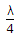# Electronics and Communication Engineering - Microwave Communication

### Exercise :: Microwave Communication - Section 6

21.

What is the numerical aperture an optical fiber when critical angle is 30°?

 A. 0.5 B. 0.704 C. 0.866 D. 0.2

Answer: Option A

Explanation:

No answer description available for this question. Let us discuss.

22.

A line of length slightly less thanand short circuited at far end behaves as

 A. inductance L B. capacitance C C. L and C in series D. L and C in parallel

Answer: Option A

Explanation:

No answer description available for this question. Let us discuss.

23.

In TE10 mode of rectangular waveguide

 A. there is one half sine wave variation along x direction and one along y direction B. there is one half sine wave variation along y direction and one along x direction C. there is one full sine wave variation along x direction and one along y direction D. none of the above

Answer: Option A

Explanation:

No answer description available for this question. Let us discuss.

24.

Reflection from the object to be measured is significant only when the wavelength is

 A. much bigger than the size of object B. much smaller than the size of object C. almost of the size of object D. either (a) or (c)

Answer: Option B

Explanation:

No answer description available for this question. Let us discuss.

25.

The speed at which axial electric field due to signal advances in a TWT is equal to

 A. speed of light B. (speed of light) (helix pitch helix circumference) C. (speed of light) (helix circumference helix pitch) D. (speed of light) (helix pitch) (helix circumference)

Answer: Option B

Explanation:

No answer description available for this question. Let us discuss.

#### Current Affairs 2021

Interview Questions and Answers